error: Content is protected !!

# PHYSICS: FORM ONE: Topic 7 - PRESSURE

TOPIC 7: PRESSURE

The Concept of Pressure
Explain the concept of pressure
Pressure is defined as the force per unit area. Mathematically, the force discussed here is the force which acts normally or perpendicularly to a unit surface area.
That is to say, pressure depends on the force applied and the surface area.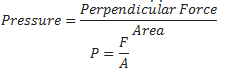The S.I Unit of Pressure
State the S.I unit of pressure
The SI unit of Pressure is Newton per square metre (N/m2). This unit is usually referred to as the Pascal (Pa).
However, due to the fact that measurement of pressure may result to very large numbers or small numbers there are other units used in measurement of pressure. These are atmosphere(atm), Millimetres of mercury (mmHg), torr and bar.
Whereby; -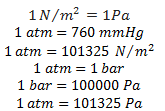Note: for a given amount of force, the smaller the area of application the greater the pressure exerted.
When a man lifts a bucket of water by its handle that is made with a thin metal, he would experience some discomfort but if the bucket was made with a thicker handle the discomfort will be much less if any.
This is because the area over which the force is applied is larger.
Dependence of Pressure on Surface of Contact
Explain dependence of pressure on surface of contact
The pressure in solid depends on the surface area of contact. A force (F) applied by a small area exerts a higher pressure as compared to when it is applied by a large surface.
This is well explained by the formula P =F/A meaning that when the value of the area is small then the value of pressure will be large and when the area is large for the same amount of force, the value of pressure will be small. That is to say, increase in area causes the decrease in pressure for the same amount of force.
That is why; -
• Feet of elephant cannot sink into soft soil even is very heavy due to large surface area over elephant feet.
• Sharp edges of knife cut easily than blunt knife because a sharp knife has a small surface area.
• A tractor and other heavy trucks works on soft ground cannot sink due to large surface area of the tyres (wide tyres).
• We feel great pain if we carry a bucket of water with a thin handle than when we use a wide (enhanced) handle.
Example 1
A block of wood that weighs 30N and measures 5m by 10m by 4m. If it was placed on a table with the largest possible area (5mx10m) in contact with table, exerts less pressure than it would when placed with its smallest possible area (5mx4m) in contact with table.
Solution
Data:
Force = 30N
Largest base area = ?
AL = 10mx5m = 50m2
P = F/A = 30N = 0.6N/30m2
Pressure = 0.6N/m2
Force (F) = 30N.
Small base are = ?
As = 5mx4m
As = 20m2
P= F/A = 30N/20M2 = 1.5 N/M2
Pressure = 1.5 N/M2
Example 2
A tip of needle has a cross- sectional area of 1x106m2. If doctor applies a force of 2N to a syringe that is connected to the needle, what is pressure is exerted at the tip of the needle?
Soln:
Data:
Area (A) = 1x106m2
Force (F) = 20N
Pressure =?
P = F/A
P = 20 = 2.0x107 N/M2
The pressure extended by the needle lip is 2.0x107N/m2
Example 3
A rectangle metal block with sides 105m by 1.0m by 1.2m rests on a horizontal surface. If the density of the metal is 7000kg/m3. Calculate the maximum and minimum pressure that the block can exerts on the surface.
(Take the weight of 1kg mass to be 10N)
Solution:
Data:
Dimension = 1.2m,1.5m,1.0m.
Density = 7000kg/m3
Maximum pressure (Pmx) = ?
Minimum pressure (Pmn) = ?
Volume = 1.2x1.5x1=18m3
Density = mass/ Volume
7000kg/m3 = mass/18
Mass = 7000x18 = 126000Kg
1kg = 10N
126000Kg = ?
F = 1260000 = 1.26 x 106
Force of metal = 1.26 x 106
A1 = 1.2 x 1.5= 18m2
A2= 1.2m2= 1.2mx1m=1.2m2
A3= 1.5 x 1m= 1.5m2
Amx = 18m2
Amin = 102m2
Pmn = F/Amx =1.26 x 106N/18M= 1.26X106/1.8 X101
Maximum pressure = 1.05x106N/m2/maximum pressure= 0.7x105N/m2

The Applications of Pressure due to Solids
Identify the applications of pressure due to solids
It is used to make different objects like screw, nails, pins, spears and arrows. This item is given sharp points to increase their penetrating power.
It helps some of living organisms for self-defence. A fish uses its sharp fins to protect itself.
When one walks on shoes with sharp pointed heels they exert greater pressure on the ground than when wearing flat shoes.
It helps in construction of railways. During the constructions of railways, wide wooden or concrete sleepers are placed below the railways tracks. This provides a larger surface area over which the weight of the train acts. This is a safety measure to train tracks.
Buildings are constructed with wide foundations to ensure that the weight of the building acts over the layer area.

A liquid will exert pressure on an immersed object as well as on the walls of the container holding it. Note that the pressure exerted by liquids is due to the weight of the liquid. Also increase in water level in liquid cause increase in pressure.
The Characteristics of Pressure in Liquids
Describe the characteristics of pressure in liquids
The pressure at any point in a liquid at rest depends on the following factors;
1. Depth of the liquid
2. Density of liquid
This can be shown from the general formula for pressure as follows;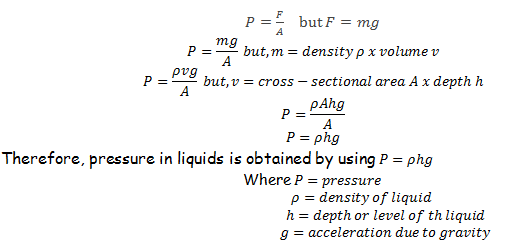Pressure in liquids has the following characteristics; -
1. Pressure in a liquid increase with depth
2. Pressure in a liquid act equally in all directions
3. Pressure in a liquid increase with increase in density of the liquid.
Number 3 explains the reason why Mercury exerts more pressure than an equal volume of water. It is simply because mercury is denser than water.
The Variation of Pressure with Depth in Liquids
Examine the variation of pressure with depth in liquids
The pressure in liquids increase with the increase in depth. Consider the cylindrical vessel containing water and with holes A, B and C punched on one of its sides.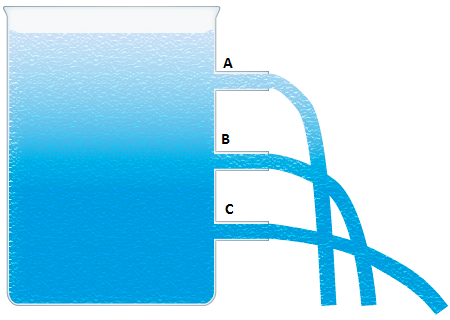Pressure of the water at hole A is small because the depth of water or length of water column from point A to the top is small. This causes water to flow at a low speed and at a small distance from the bottom of the vessel.
Pressure of the water at hole B is large than at A because the depth of water or length of water column from point B to the top is somehow larger. This causes water to flow at a greater speed and at a larger distance from the bottom of the vessel.
Pressure of the water at hole C is large because the depth of water or length of water column from point C to the top is very large. This causes water to flow at a high speed and at a small distance from the bottom of the vessel.
Problems Involving Pressure in Liquids
Solve problems involving Pressure in Liquids
Example 4
A cube of side 2cm is completely submerged in water so that the bottom of the cube is at a depth of 10cm. use g=10m/s2 and s=1000kg/m3
1. What is different between the pressure on the bottom of the cable and the pressure on its tap?
2. Determine the difference in the force on the top and bottom.
3. What is the weight of the water displaced by the cube?
Solution;
Data.
Depth = 10cm = 0.1m
s = 1000kg/m3
g = 10m/s
h= 8cm= 0.08
formular
p= sgh
p=1000kg/m3x10m/s2x0.1m=1000N/m2
At the h= 0.08m p= 1000x10x0.08= 800N/m2
Change in pressure = 1000N/m =200N/m2
So that different in pressure between the bottom the top is 200N/m2
Pressure = Force/Area = P= F/A
Area =L2= (10.02)2= 0.0004m2
Distance in water 200N/m2x0.0004m2
This is the upthrust acting on the cube
Water displaced by the cube in weight= volume of the cube
Volume = l3 (2cm) 3 = 8cm3
For water, 1cm3= 1g 50.8cm3 = 8g
Weight of displaced water
mass of displaced water/100=8g = 0.08N
Example 5
Calculate the pressure at the bottom of tank of water 15m deep due to the water above it is (s=1000kg/m3).
Solution:
Data:
Height =15m
S=1000kg/m3
g= 10m/s2
Formula
Pressure in liquid sgh
= 1000x10x15=150000N/M2

The Principle of a Hydraulic Pressure
Explain the principle of a hydraulic pressure
The hydraulic press is the simple device that works under the Pascal’s principle of transmission of pressure. It contains a small piston on one side and a large piston on the other side.
A force is applied to a small piston and pressure is transmitted to the large piston through the fluid inside it.
According to Pascal’s principle the pressure is transmitted equally to the large piston which is then converted to a large amount of force.
The Pascal’s principle states that; - “Any external pressure applied to the surface of an enclosed liquid will be transmitted equally throughout liquid.”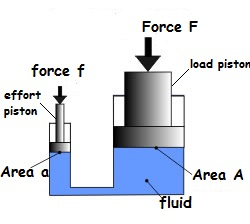The Hydraulic press
From Pascal’s Principle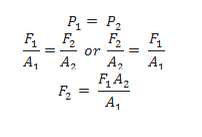Example 6
The pistons of a hydraulic press have their areas given as 3.0x10-4m2 and 2x102m2 respectively. If the smaller piston is pushed down with a force of 12N, what is the force required to push the larger piston?
Soln:
Data:
F1 = 120N, A2= 2X102m2
A2 = 3X104m2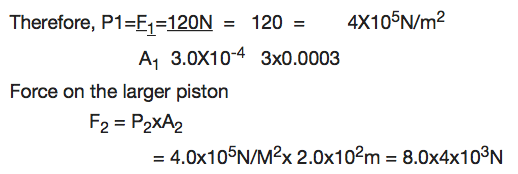Application of Hydraulic Press
Measure pressure of a liquid
Hydraulic press is used in industries to express bulk items.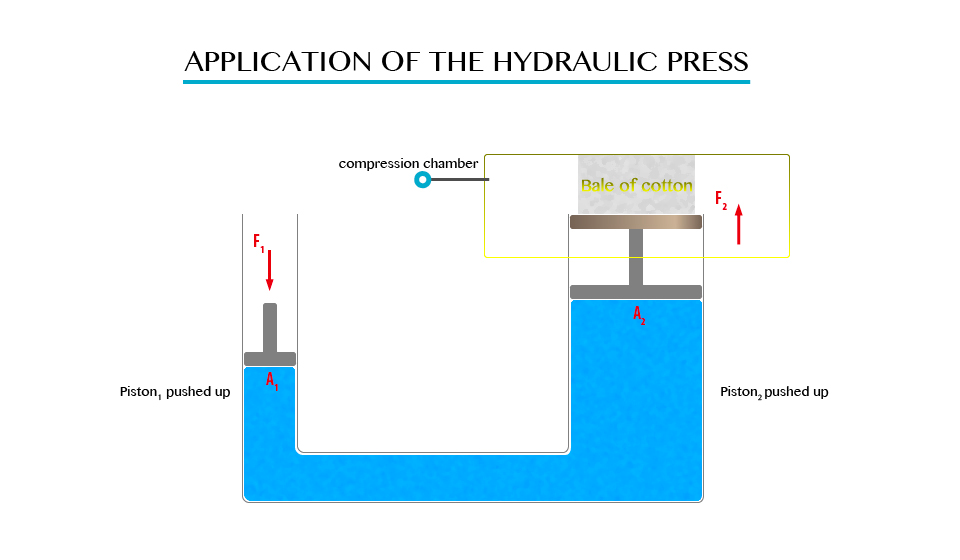Hydraulic brake system
When pressure is applied to the brake pedal, it pushes the piston in the master cylinder forward creating a pressure in the brake fluid. This pressure is transferred to the slave cylinders where it is multiplied and pushes the brake shoes against the brake dram that is attached to the wheel of the vehicle.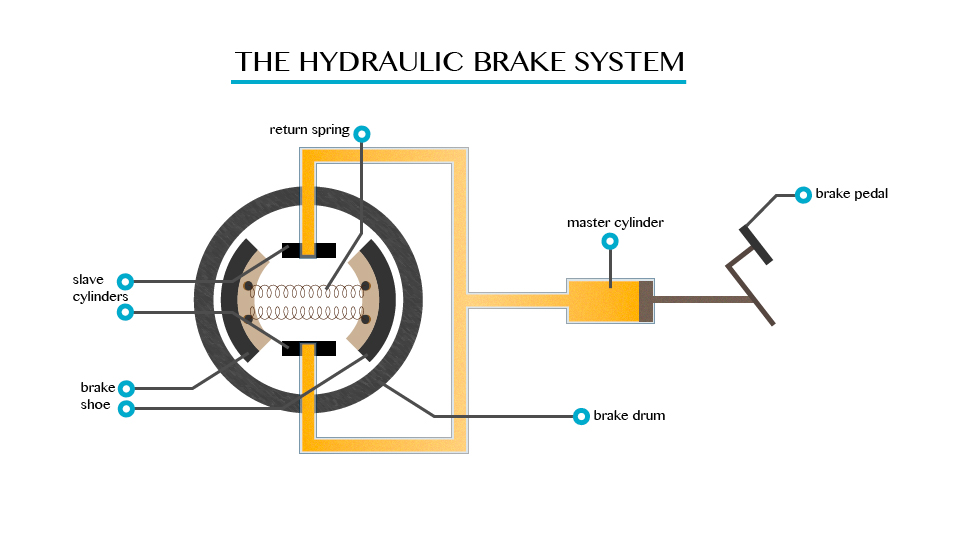It is used in industries in the forming of metals.
Since the hydraulic press act like a lift, it is used for lifting heavy loads.
Measuring the pressure of liquids using a manometer. Manometer is the device for measuring pressure (commonly gas).

The Existence of Atmospheric Pressure
Describe the existence of atmospheric pressure
Atmospheric pressure is as a result of the weight of this layer of gases, the act at atmospheric pressure is surrounded the layer of air consisting of a mixture of gases.
Note. The atmospheric pressure on the earth’s surface and objects on the earth is a approximately 1.01x105N/m2.
The experiment which demonstrate the existence of atmospheric pressure.
Umbler experiment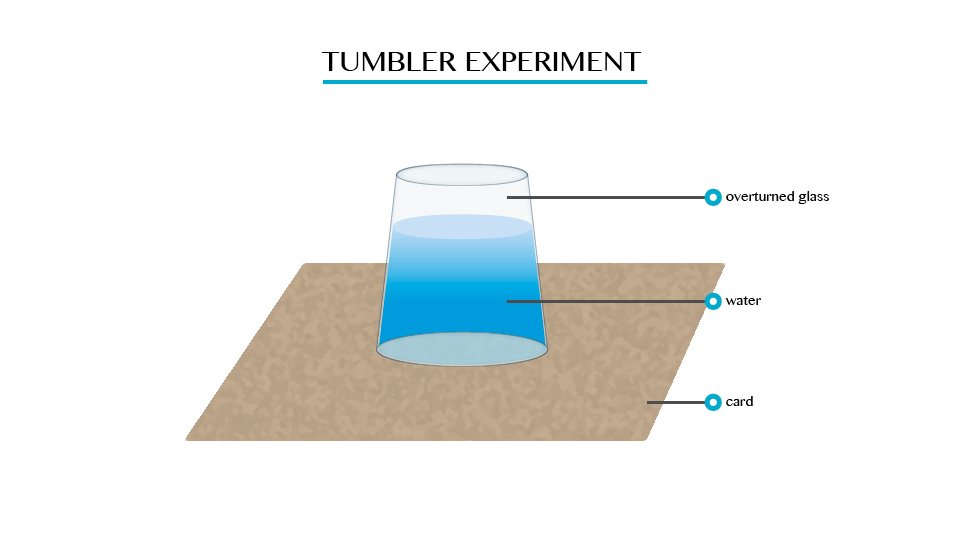When you fill a glass timber with cord and gentle turned upside down. It will be seen that water will not poured down.
Plunger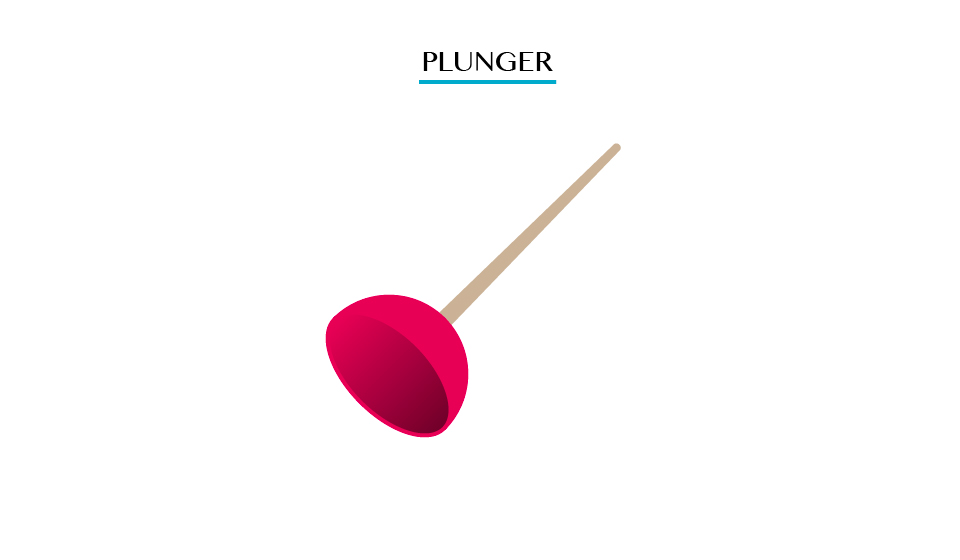Pulling the plunger is not easy. This is so because all the air is squeezed out from the table when the surrounding pressure being high the plunger sticks.
Crushed bottle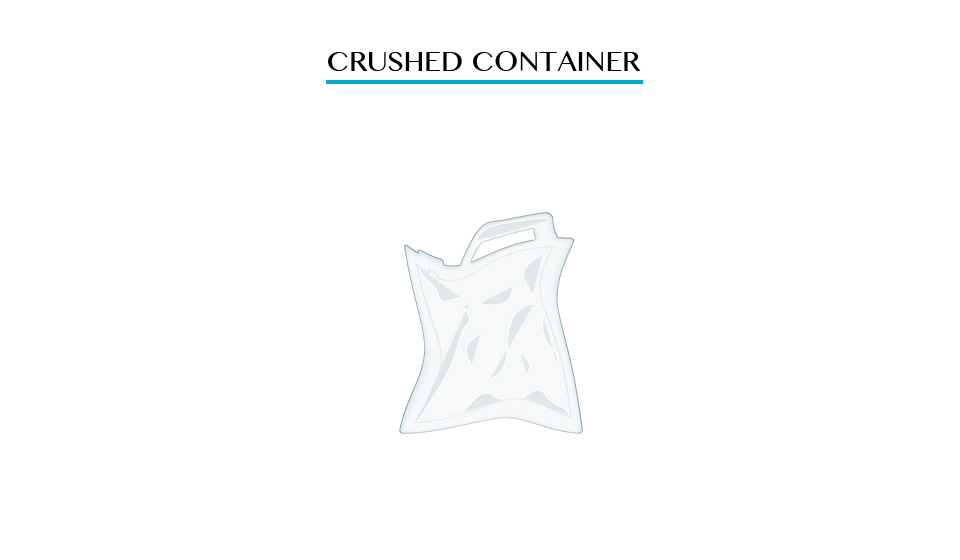When putting hot water in, the bottle is cooled under cold water the steam condenses; leaving partial vacuum inside the bottle consequently the greater atmospheric pressure outside the bottle crushes it in wards.

Applications of Atmospheric Pressure
Identify the applications of atmospheric pressure
A siphon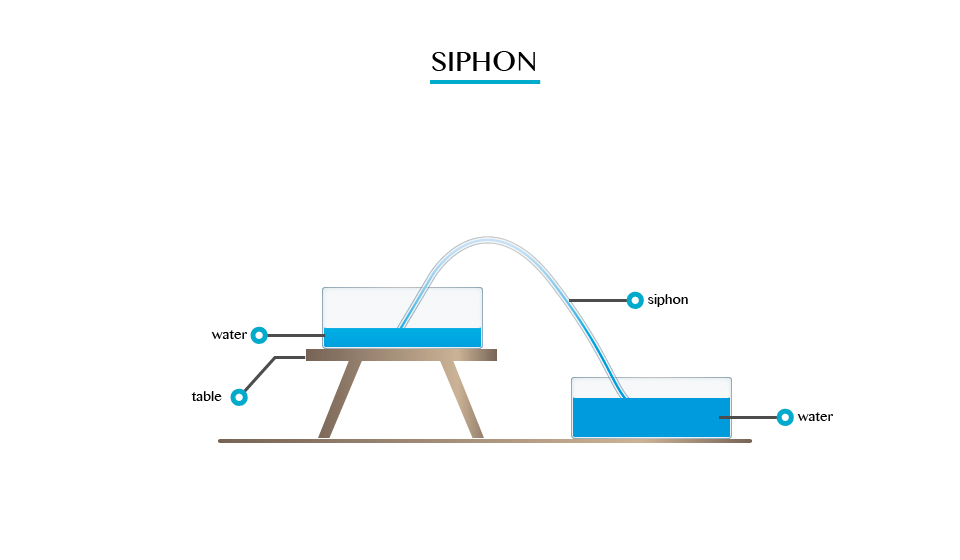A siphon is applied in areas and devices that we use everyday
1. It is used in the toilet flushing cisterns (chain and ball tank). The flush is triggered by a handle that operates a simple diaphragm like piston pump that lifts enough water.
2. It is used in special rain gauges called siphon rain gauge which are able to automatically drain out excess water.
3. A siphon cup is a reservoir attached to a gum.
4. It is used is some drainage systems to drain water to one point.
The lift pump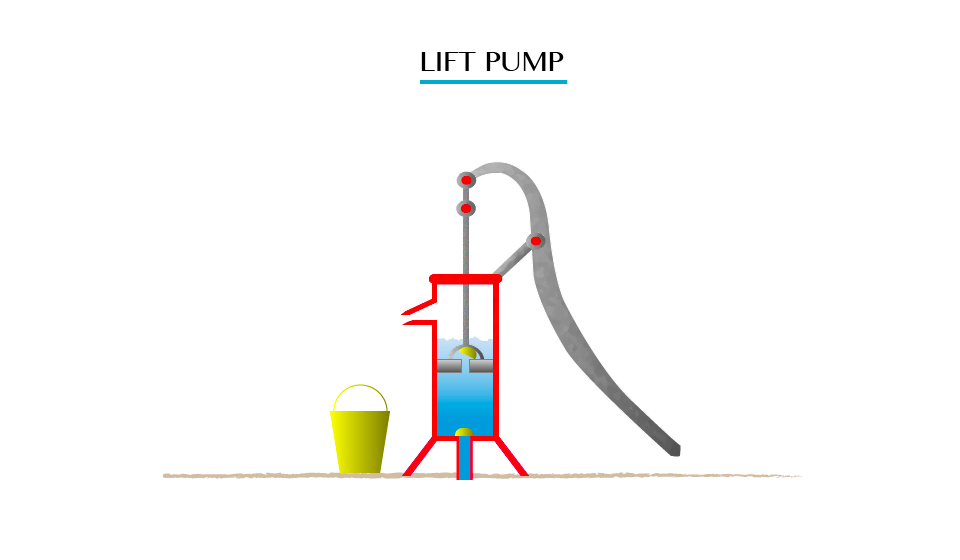A lift pump is used to raise water from underground sources. This is a pump that is used to lift the liquid, rather than force liquid up.
A syringe is a simple piston pump that lifts a tube. The plunger that lifts can be pulled and pushed through inside a cylindrical tube or barrel. This enables the syringe to take in or expel fluid through the opening (nozzle) at the end of the tube.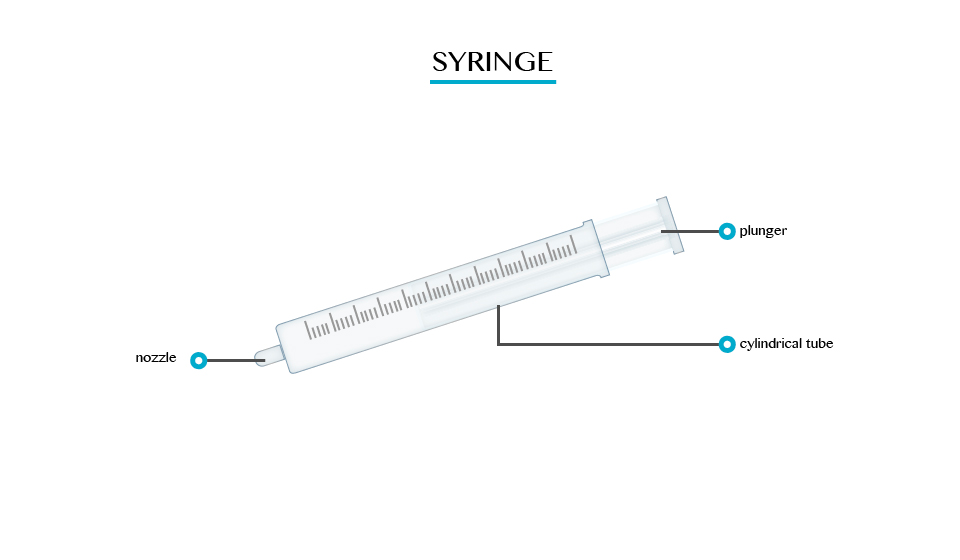Uses of syringe:
1. They can be fitted with hypodermic needles and used to administer injections.
2. They are used to measure liquids and gases in a laboratory.
3. They are used to apply certain compounds such as a glue or lubricant.
Bicycle pump
This is a type of force pump that consists of a hollow metal cylinder and a movable piston.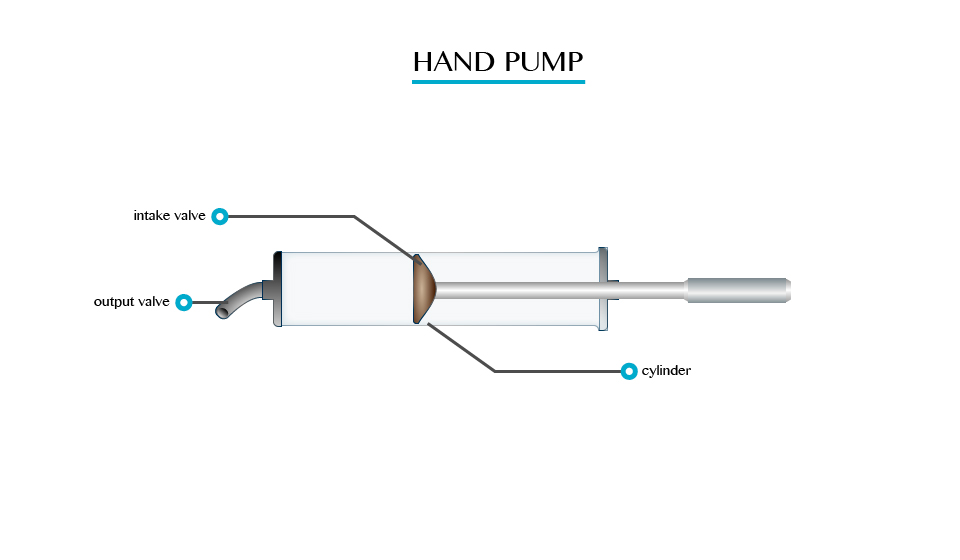Meassuring Atmospheric Pressure
Measure atmospheric pressure
Atmospheric pressure is measured by using barometer;
Types of barometer:
1. The simple barometer
2. Fortin barometer
3. Aneroid barometer
This is a type of force pump that consists of a hollow metal cylinder and a movable piston.
A simple barometer is the most fundamental of the other types of barometer. The barometric liquid used is mercury.
A simple barometer consists of a hard glass tube closed at one end.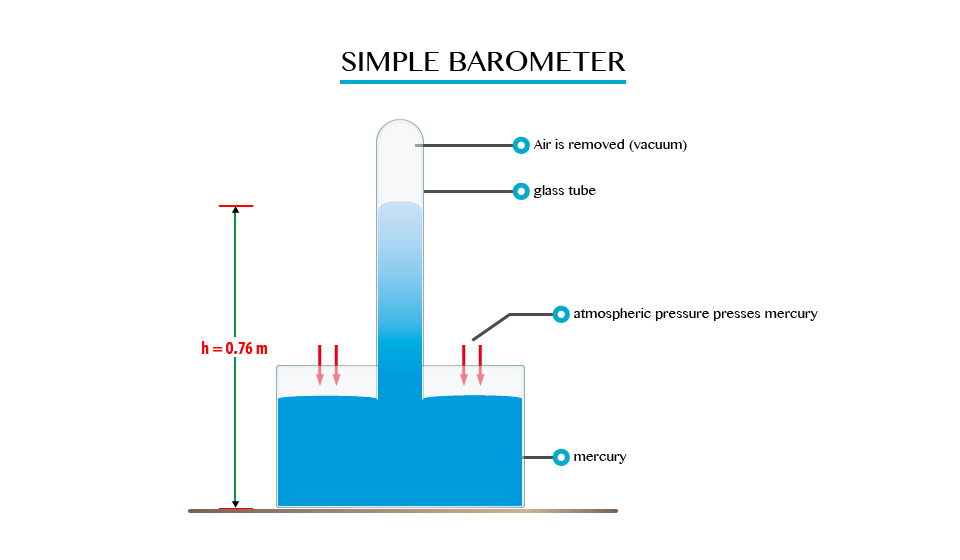Fortin barometer
A Fortin barometer is a modified simple barometer. It consists of an inverted tube closed at its upper end with the lower open end immersed in a reserve of mercury. The atmospheric pressure is measured in terms of the height of the column of mercury.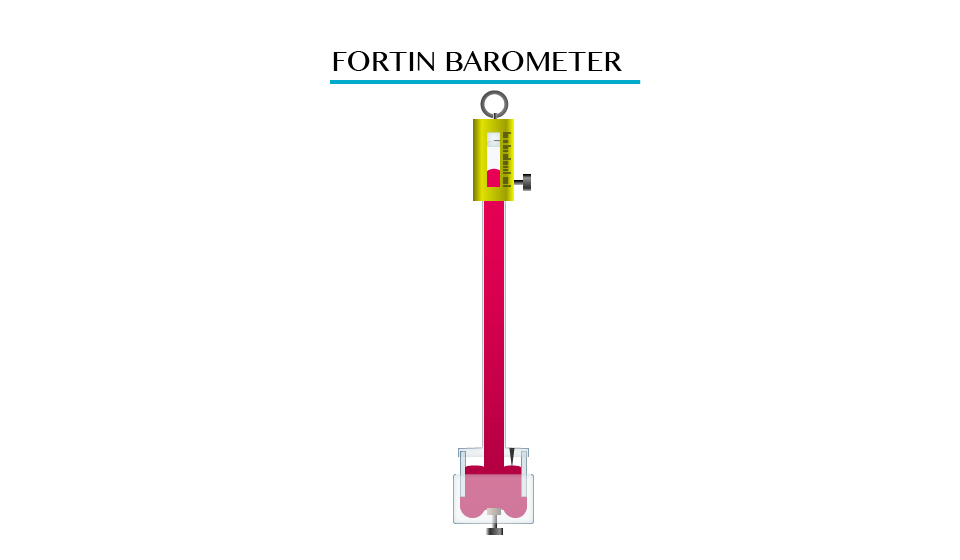1.